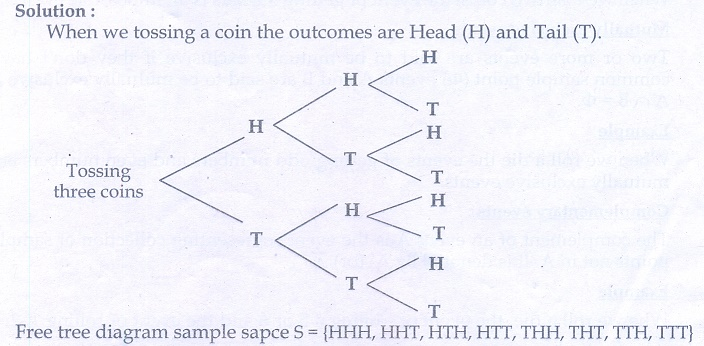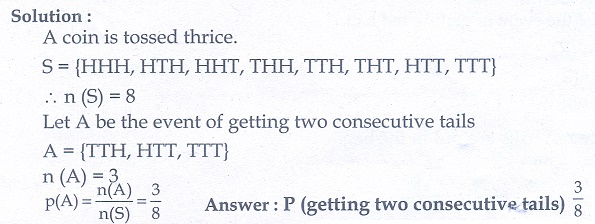Home | | Maths 10th Std | Exercise 8.3: Probability

# Exercise 8.3: Probability

Maths Book back answers and solution for Exercise questions - Mathematics : Probability: Exercise Problem Questions with Answer

### Exercise 8.3

1. Write the sample space for tossing three coins using tree diagram.2. Write the sample space for selecting two balls from a bag containing 6 balls numbered 1 to 6 (using tree diagram).3. If A is an event of a random experiment such that P(A) : P () =17:15 and n(S)=640 then find (i) P () (ii) n(A).3.B4. A coin is tossed thrice. What is the probability of getting two consecutive tails?5. At a fete, cards bearing numbers 1 to 1000, one number on one card are put in a box. Each player selects one card at random and that card is not replaced. If the selected card has a perfect square number greater than 500, the player wins a prize. What is the probability that (i) the first player wins a prize (ii) the second player wins a prize, if the first has won?6. A bag contains 12 blue balls and x red balls. If one ball is drawn at random (i) what is the probability that it will be a red ball? (ii) If 8 more red balls are put in the bag, and if the probability of drawing a red ball will be twice that of the probability in (i), then find x.7. Two unbiased dice are rolled once. Find the probability of getting

(i) a doublet (equal numbers on both dice)

(ii) the product as a prime number

(iii) the sum as a prime number

(iv) the sum as 18. Three fair coins are tossed together. Find the probability of getting

(ii) atleast one tail

(iv) atmost two tails9. Two dice are numbered 1,2,3,4,5,6 and 1,1,2,2,3,3 respectively. They are rolled and the sum of the numbers on them is noted. Find the probability of getting each sum from 2 to 9 separately.10. A bag contains 5 red balls, 6 white balls, 7 green balls, 8 black balls. One ball is drawn at random from the bag. Find the probability that the ball drawn is

(i) White

(ii) black or red

(iii) not white

(iv) neither white nor black11. In a box there are 20 non-defective and some defective bulbs. If the probability that a bulb selected at random from the box found to be defective is 3/8 then, find the number of defective bulbs.12. The king and queen of diamonds, queen and jack of hearts, jack and king of spades are removed from a deck of 52 playing cards and then well shuffled. Now one card is drawn at random from the remaining cards. Determine the probability that the card is

(i) a clavor

(ii) a queen of red card

(iii) a king of black card13. Some boys are playing a game, in which the stone thrown by them landing in a circular region (given in the figure) is considered as win and landing other than the circular region is considered as loss. What is the probability to win the game?14. Two customers Priya and Amuthan are visiting a particular shop in the same week (Monday to Saturday). Each is equally likely to visit the shop on any one day as on another day. What is the probability that both will visit the shop on

(i) the same day

(ii) different days

(iii) consecutive days?15. In a game, the entry fee is 150. The game consists of tossing a coin 3 times. Dhana bought a ticket for entry. If one or two heads shows she gets her entry fee back. If she throwns 3 heads she receives double the entry fees. Otherwise she will lose. Find the probability that she (i) gets double entry fee (ii) just gets her entry fee (iii) loses the entry fee.